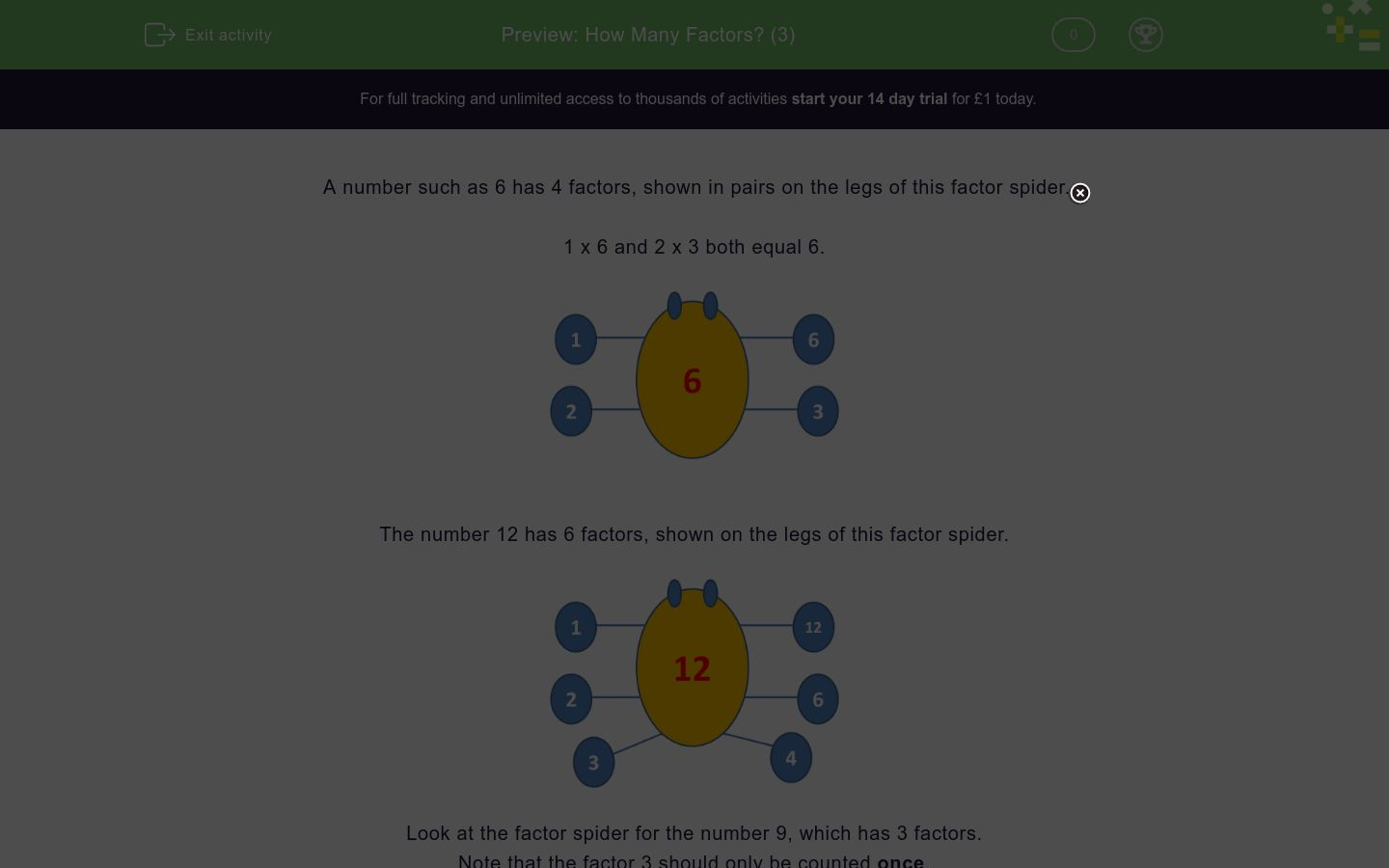# How Many Factors? (3)

In this worksheet, students state how many factors a number has by looking at its "factor spider"!Key stage:  KS 2

Curriculum topic:   Number: Multiplication and Division

Curriculum subtopic:   Identify Multiples and Factors

Difficulty level:### QUESTION 1 of 10

A number such as 6 has 4 factors, shown in pairs on the legs of this factor spider.

1 x 6 and 2 x 3 both equal 6.The number 12 has 6 factors, shown on the legs of this factor spider.Look at the factor spider for the number 9, which has 3 factors.

Note that the factor 3 should only be counted once.Want to understand this further and learn how this links to other topics in maths?

Why not watch this video?

How many factors does the following number have?

16

How many factors does the following number have?

17

How many factors does the following number have?

48

How many factors does the following number have?

49

How many factors does the following number have?

50

How many factors does the following number have?

51

How many factors does the following number have?

27

How many factors does the following number have?

61

How many factors does the following number have?

47

How many factors does the following number have?

72

• Question 1

How many factors does the following number have?

16

5
EDDIE SAYS
16: 1, 2, 4, 8, 16
• Question 2

How many factors does the following number have?

17

2
EDDIE SAYS
17: 1, 17
• Question 3

How many factors does the following number have?

48

10
EDDIE SAYS
48: 1, 2, 3, 4, 6, 8, 12, 16, 24, 48
• Question 4

How many factors does the following number have?

49

3
EDDIE SAYS
49: 1, 7, 49
• Question 5

How many factors does the following number have?

50

6
EDDIE SAYS
50: 1, 2, 5, 10, 25, 50
• Question 6

How many factors does the following number have?

51

4
EDDIE SAYS
51 is divisible by 3 51: 1, 3, 17, 51
• Question 7

How many factors does the following number have?

27

4
EDDIE SAYS
27: 1, 3, 9, 27
• Question 8

How many factors does the following number have?

61

2
EDDIE SAYS
Prime 61: 1, 61
• Question 9

How many factors does the following number have?

47

2
EDDIE SAYS
Prime 47: 1, 47
• Question 10

How many factors does the following number have?

72

12
EDDIE SAYS
72: 1, 2, 3, 4, 6, 8, 9, 12, 18, 24, 36, 72
---- OR ----

Sign up for a £1 trial so you can track and measure your child's progress on this activity.

### What is EdPlace?

We're your National Curriculum aligned online education content provider helping each child succeed in English, maths and science from year 1 to GCSE. With an EdPlace account you’ll be able to track and measure progress, helping each child achieve their best. We build confidence and attainment by personalising each child’s learning at a level that suits them.

Get started